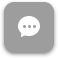Call Now 855-300-1469

# CIS 3315 Decision Support Systems

#### Course Description

Provides the knowledge and skills necessary to create applications, collect data, and manage databases. Course includes the theory of decisions and decision analysis, including probability and risk-taking. Uses commercial decision support and graphics software to prepare business packages such as forecasting, linear programming, and decision trees.

#### Course Objectives

Upon completion of this course, students should be able to

• Demonstrate an understanding of the theory of decisions and decision analysis including probability, decisions under uncertainty, risk taking, and real-world problems
• Describe and understand the concept of decision support systems including collection of data, database management, modeling, group and organizational decision support systems, executive information systems, and expert systems
• Utilize commercial decision support and graphic presentation software packages to prepare typical business decision packages utilizing methodologies such as forecasting, linear programming, and decision trees

### Week 1

##### Lecture: Introduction to Managerial Decision Making

Outcomes

• Define decision model and describe its importance
• Describe two types of decision models: deterministic and probabilistic models
• Follow steps involved in developing decision models in practice
• Use spreadsheets in developing decision models
• Discuss possible problems in developing decision models

### Week 2

##### Module: Linear Programming Models: Model Formulation and Computer Solution

Outcomes

• Describe basic assumptions and properties of linear programming (LP)
• Formulate a LP model
• Set up LP problems on a spreadsheet and solve them using Excel Solver

### Week 3

##### Module: Linear Programming Modeling Applications: Computer Analyses in Excel

Outcomes

• Create a two-dimensional array
• Store data in a two-dimensional array
• Search a two-dimensional array
• Sum the values in a two-dimensional array

### Week 4

##### Lecture: Linear Programming: Sensitivity Analysis

Outcomes

• Analyze the impact of changes in objective function coefficients, right-hand-side values, and constraint coefficients on optimal solution of a linear programming problem
• Generate answer and sensitivity reports using Excel Solver
• Interpret information in answer and sensitivity reports

### Week 5

##### Lecture: Transportation, Assignment, and Network Models

Outcomes

• Structure special LP network flow models
• Set up and solve transportation models using Excel Solver to determine minimum cost shipping routes in a network
• Review transshipment, maximal-flow, shortest-path, and minimal-spanning tree network models

### Week 6

##### Lecture: Project Management

Outcomes

• Formulate integer programming (IP) models
• Set up and solve IP models using Excel Solver
• Differentiate between general integer and binary integer variables
• Use binary integer variables in formulating problems involving fixed (or setup) costs
• Plan, monitor, and control projects using PERT/CPM
• Determine earliest start, earliest finish, latest start, latest finish, and slack times for each activity
• Recognize the important role of software such as Microsoft Project in project management

### Week 7

##### Lecture: Queuing Models

Outcomes

• Describe different types of decision-making environments
• Make decisions under uncertainty when probabilities are not known
• Make decisions under risk when probabilities are known
• Use Excel for problems involving decision tables
• Develop accurate and useful decision trees
• Discuss trade-off curves for cost of waiting time and cost of service
• Recognize three parts of queuing system: arrival population, queue itself, and service facility
• Describe basic queuing system configurations
• Describe assumptions of common queuing models

### Week 8

##### Lecture: Inventory Control Methods

Outcomes

• Determine when to use various types of forecasting models
• Compute a variety of forecasting error measures
• Describe Delphi and qualitative decision-making approaches
• Compute moving and weighted moving averages, and exponential smoothing time-series models
• Analyze trends and seasonality in time-series data
• Recognize the importance of inventory control
• Use economic order quantity (EOQ) model to determine how much to order
• Compute reorder point (ROP) in determining when to order more inventory
• Use EOQ with noninstantaneous receipt model to determine how much to order or produce

The course description, objectives and learning outcomes are subject to change without notice based on enhancements made to the course.